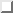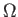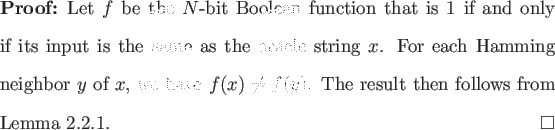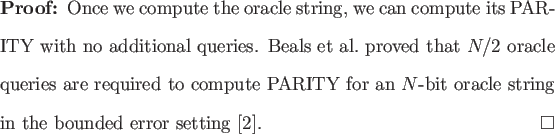Next: Singleton Functions Up: Lower Oracle Query Bounds Previous: Application to Generalized XOR   Contents

# Determining the Oracle String

Consider the problem of determining the oracle string. We prove two lower bounds for this problem, one through Lemma, and another by using a known lower bound for PARITY.

Theorem 2.3.1() oracle queries are required to determine an N-bit oracle string in the bounded error setting.In the classical case N oracle queries are required to determine the oracle string. If the lower bound of() oracle queries were asymptotically tight, then there would be quadratic improvement over the classical case for this problem. This seems plausible in light of Grover's algorithm. However,(N) lower bounds are known for Boolean functions in the quantum oracle model, we can thus infer that Theorem 2.3.1 is not asymptotically tight.

Theorem 2.3.2   N/2 oracle queries are required to determine an N-bit oracle string in the bounded error setting.We now see an apparent limitation of Ambainis' Theorem; the result attained was quadratically worse than the asymptotically tight lower bound. We will show in the next section that the weak lower bound in Theorem 2.3.1 is indeed the best that can be attained through application of Ambainis' Theorem.Next: Singleton Functions Up: Lower Oracle Query Bounds Previous: Application to Generalized XOR   Contents
Matthew Hayward Lower Query Bounds in the Quantum Oracle Model GitHub Repository